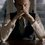# Help me!

I need help in following things -

There had been 2 problems I had been trying to guess the recurrence relation but have been unsuccessful.

1. number of different regions formed when $n$ mutually intersecting planes are drawn in 3-D space such that no four planes intersect at a common point and no two have parallel intersection lines in a 3rd plane.

2. number of regions into which a convex $n$-gon is divided by all its diagonals.

I would love to have a detailed explanation on how to find the recurrence relation - is it all about inspection, in these types of geometry problems?

One more doubt is related to mechanics. I was reading David Morin and he explains about a "slick neat trick" of finding the moment of inertia. The method is about "scaling, using $I$'s definition and using parallel axis theorem". It seemed quite helpful, in primarily his fractal triangle problem. But I couldn't understand the first step exactly i.e. scaling. Any help on this will be appreciated.

I was looking up to entropy. I understand that the entropy is first of all the proportionality constant of heat and thermodynamic temperature and it remains conserved in a reversible process and that in irreversible, it should always increase and all that. But the one thing I didn't understand is how this relates t disorder.Note by Kartik Sharma
5 years, 9 months ago

This discussion board is a place to discuss our Daily Challenges and the math and science related to those challenges. Explanations are more than just a solution — they should explain the steps and thinking strategies that you used to obtain the solution. Comments should further the discussion of math and science.

When posting on Brilliant:

• Use the emojis to react to an explanation, whether you're congratulating a job well done , or just really confused .
• Ask specific questions about the challenge or the steps in somebody's explanation. Well-posed questions can add a lot to the discussion, but posting "I don't understand!" doesn't help anyone.
• Try to contribute something new to the discussion, whether it is an extension, generalization or other idea related to the challenge.

MarkdownAppears as
*italics* or _italics_ italics
**bold** or __bold__ bold
- bulleted- list
• bulleted
• list
1. numbered2. list
1. numbered
2. list
Note: you must add a full line of space before and after lists for them to show up correctly
paragraph 1paragraph 2

paragraph 1

paragraph 2

[example link](https://brilliant.org)example link
> This is a quote
This is a quote
    # I indented these lines
# 4 spaces, and now they show
# up as a code block.

print "hello world"
# I indented these lines
# 4 spaces, and now they show
# up as a code block.

print "hello world"
MathAppears as
Remember to wrap math in $$ ... $$ or $ ... $ to ensure proper formatting.
2 \times 3 $2 \times 3$
2^{34} $2^{34}$
a_{i-1} $a_{i-1}$
\frac{2}{3} $\frac{2}{3}$
\sqrt{2} $\sqrt{2}$
\sum_{i=1}^3 $\sum_{i=1}^3$
\sin \theta $\sin \theta$
\boxed{123} $\boxed{123}$

Sort by:

1. Have you seen the 2-D version of this problem? If yes, duplicate it. If no, think about it. If you're stuck, try the 1-D version.

2. It depends on whether the n-gon is regular, convex, etc.

Staff - 5 years, 9 months ago

R u talking about this right? If yes, it is clearly shown the application of parallel axis theorem. Easy way to find out the moment of inertia through any axis passing through the centre of mass is to first find the moment of inertia of the centre of mass and add it to $md^2$, where m is mass and d is distance between centre of mass and the axis. It is quite evident that Morin has used the same method.

- 5 years, 9 months ago

I am not saying how to find the answer of fractal triangle problem (although this is not the problem's solution as such, because in the problem the axis is not perpendicular to the plane, it is along the altitude). But yeah, you got it right, I am talking quite the same thing. In particular, what I am asking is to give insights into why the 1st step(scaling argument) as the image shows, makes sense. Okay, I can easily go on with the steps given but it does not make sense to me. It would make sense only when I use the integral which however is to be leaved in the "trick".

And no, I am not saying anything about parallel - axis theorem. In your image only, it is used at the 3rd step only and I am talking about the 1st step.

- 5 years, 9 months ago